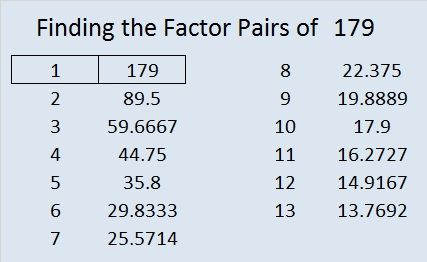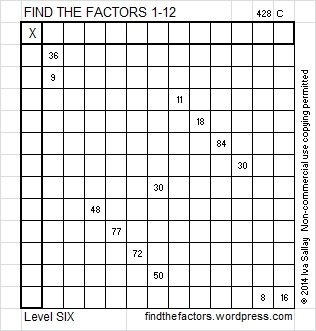# 179 and Level 6

• 179 is a prime number.
• Prime factorization: 179 is prime.
• The exponent of prime number 179 is 1. Adding 1 to that exponent we get (1 + 1) = 2. Therefore 179 has exactly 2 factors.
• Factors of 179: 1, 179
• Factor pairs: 179 = 1 x 179
• 179 has no square factors that allow its square root to be simplified. √179 ≈ 13.379088How do we know that 179 is a prime number? If 179 were not a prime number, then it would be divisible by at least one prime number less than or equal to √179 ≈ 13.4. Since 179 cannot be divided evenly by 2, 3, 5, 7, 11, or 13, we know that 179 is a prime number.Excel file of puzzles and previous week’s factor solutions: 12 Factors 2014-07-14